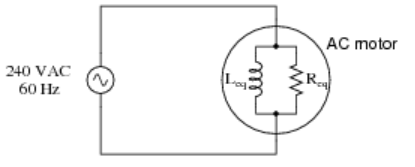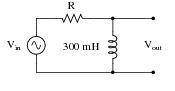### RL circuits (AC)

#### Problem:

A large AC electric motor under load can be considered as a parallel combination of resistance and inductance.  If the equivalent resistance is 20 Ω and the inductance is 238 mH, calculate the rms current delivered by the 60 Hz, Vrms = 240 V AC power supply.Solution:

• Concepts:
AC circuits
• Reasoning:
We are analyzing a parallel RL circuit.
• Details of the calculation:
Irms = Vrms/|Z|.
Z = RZL/(R + ZL) = (ω2L2R + iωLR2)/(R2 + ω2L2)
= ωLR(R2 + ω2L2)½/(R2 + ω2L2)exp(iφ) = ωLR/(R2 + ω2L2)½exp(iφ) = |Z|exp(iφ).
|Z| = 19.52 Ω.
Irms = Vrms/|Z| = (240 V)/(19.52 Ω) = 12.3 A.

#### Problem:

In an AC circuit, a filter circuit or filter removes unwanted frequency components from an input.  A passive filter consists of a circuit with at least one reactive component.  A filter with a single reactive component, either a capacitor or an inductor, is called a first-order filter.  Filters are described according to their behavior.  A low-pass filter will pass all signals below a specific frequency, but will attenuate or block signals of a higher frequency.  A high-pass filter will do just the opposite.  The boundary line is somewhat arbitrary since there exists a "transition zone".  The cutoff frequency is defined as the frequency at which |Vout| = 2|Vin|.
Inspect the filter shown below.  Identify what type of filter this circuit is, and calculate the size of resistor necessary to give it a cutoff frequency of 3 kHz:Solution:

• Concepts:
AC circuits
• Reasoning:
We have a series RL circuit.
• Details of the calculation:
Z = R + iωL.
We want Vout = Vin ZL/Z = Vinexp(iφ)ωL/(R2 + (ωL)2)½ = (1/√2)Vinexp(iφ).
We need R  = ωL = 2π*3*103*3*10-1 Ω = 5.65 kΩ.
This is a high-pass filter.